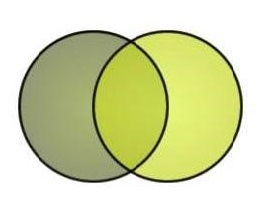# atomic orbital

n=1

A Probability Cloud......

a: An atomic orbital ~
b: fuzzy cloud

What: "An atomic orbital is like a fuzzy cloud in which the density at a given point is proportional to the probability of finding the electron at that point"

Useful?
Writer: Not Stated
LCC: